Home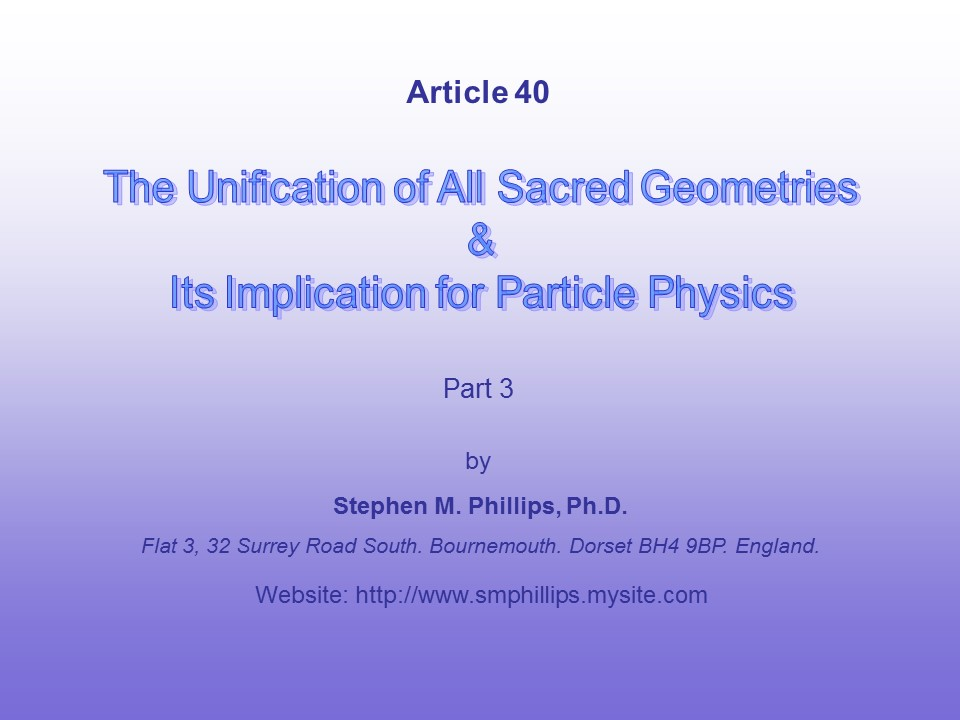1

Figure 1

The quartic equation:

x3y + y3z + z3x = 0

was studied by the mathematician Felix Klein. He showed that its Riemann surface is mapped onto itself (hence “automorphisms”) by 168 analytic transformations. These symmetries are mapped onto a surface of genus 3, i.e., the 3-torus. In fact, this is the maximum number of symmetries for a surface of this genus. This Riemann surface can be represented in the hyperbolic plane by the Klein Configuration. For the {7,3} tiling, it has 168 red, hyperbolic triangles denoting the 168 automorphisms and 168 blue triangles denoting the 168 anti-automorphisms. The 168 red or blue triangles are grouped 24 to each o seven sectors, forming 24 heptagons. Three heptagons meet at each of its 56 vertices. Each sector has two slices. Each of the 14 slices shown numbered has 12 red triangles and 12 blue triangles. The 168 automorphisms belong to the group PSL(2,7). The 168 automorphisms and 168 anti-automorphisms belong to the double-cover group SL(2,7).

2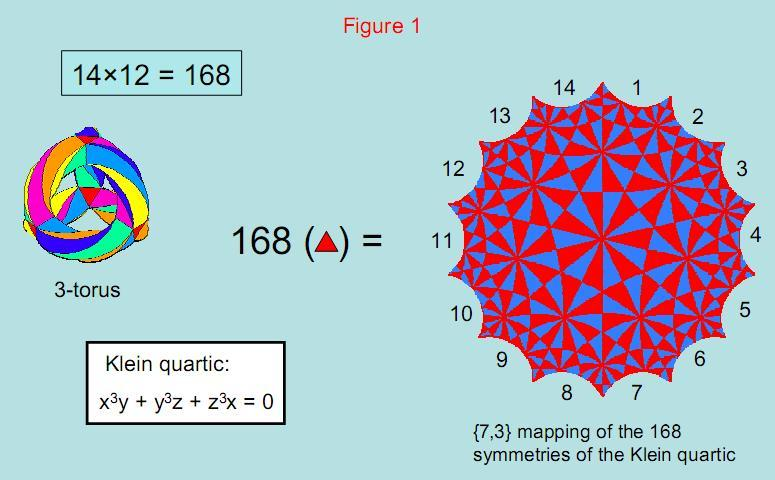The Klein configuration is the mapping on the hyperbolic plane of the 168 automorphisms (red hyperbolic triangles) of the Klein quartic. The 168 blue triangles denote its 168 anti-automorphisms. 3

Figure 2

In their book Occult Chemistry, published in 1908, Annie Besant and C.W. Leadbeater recorded their observations of what they believed were atoms of all the elements. The basic unit of matter, or “ultimate physical atom” (UPA), has two varieties, one the mirror image of the other. In the positive type, the ten closed curves, or “whorls,” spiral clockwise 2½ times around the axis about which the particle spins, then wind back 2½ times in a narrower helix back to the top of the particle. Each of the ten helices is a closed curve with 1680 turns. There are 336 turns in each of the five revolutions of each whorl, that is, 168 turns in a half-revolution. The ten whorls make 3360 turns in each revolution. The author proved that these particles are constituents of up and down quarks and has interpreted them as E8×E8' heterotic superstrings.

4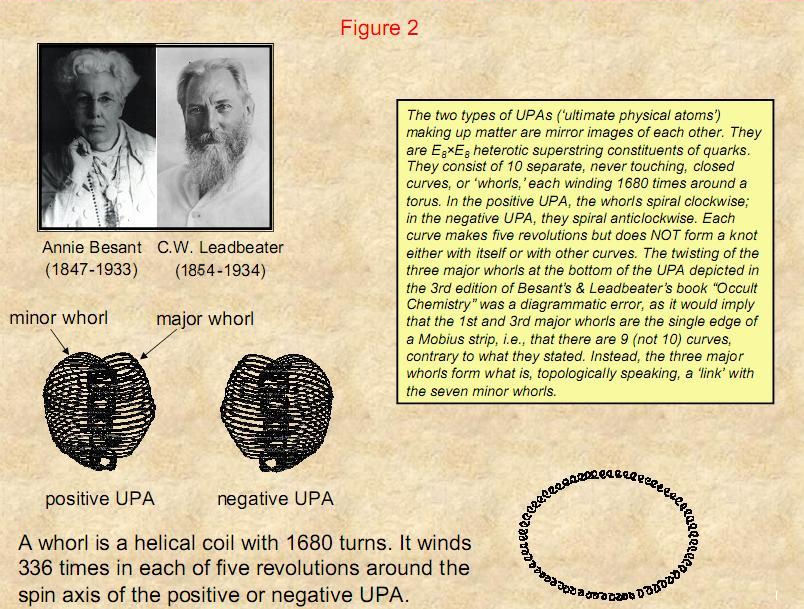5

Figure 3

The numbers 84, 168 and 336 appear in the Sri Yantra. Its 42 triangles have 84 vertices (black yods), 84 (red) hexagonal yods on the edges of its 14 outermost triangles and 168 (blue) hexagonal yods on the edges of the 28 triangles in its first three layers. 336 yods therefore line its 42 triangles, delineating its shape. 168 yods mark out each half. The Sri Yantra therefore represents the number of turns in one revolution of each helical whorl of the UPA. If one assigns the number 10 (the Pythagorean Decad symbolized by the tetractys) to each yod, the Sri Yantra generates the number 3360. This is the number of turns in one revolution of all ten whorls of the UPA. If one assigns the number value 50 of the Godname ELOHIM to each yod, the Sri Yantra generates the 16800 turns in all ten whorls of the UPA, a heterotic superstring. Created out of the number value of ELOHIM, the Godname of the third member of the Supernal Triad, the Sri Yantra generates the form of the fundamental subatomic particle making up the atoms of the elements. The Godname YAHWEH of the second member of the Supernal Triad with number value 26 prescribes the 26 dimensions of space-time from which the 10-dimensional superstring emerges.

6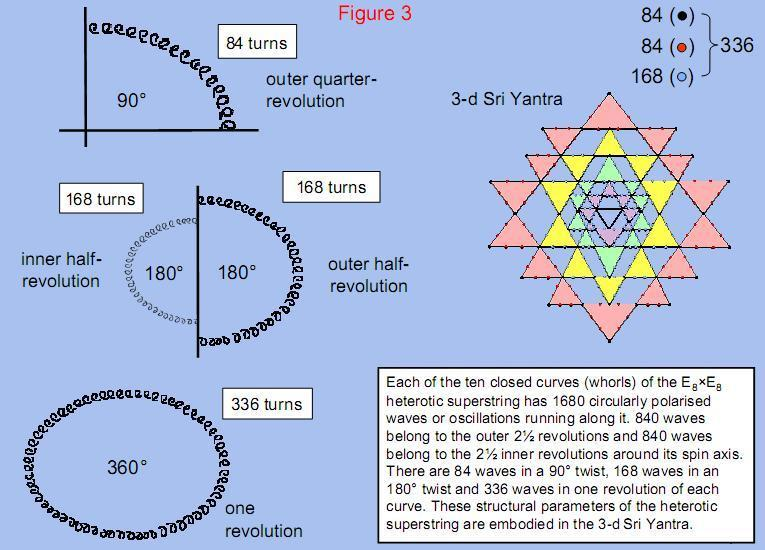7

Figure 4

The I Ching table, used for thousands of years by the Chinese for purposes of divination, is an 8×8 array of 64 hexagrams, each a pair of trigrams. There are eight hexagrams in its diagonal and 28 hexagrams (56 trigrams with 168 lines and broken lines) on either side of it. The table is equivalent to the Klein Configuration because the 168 red lines and broken lines of the 56 trigrams on one side of the diagonal correspond to the 168 red hyperbolic triangles denoting the 168 automorphisms, whilst the 168 blue lines and broken lines of the 56 trigrams on the other side of the diagonal correspond to the 168 blue hyperbolic triangles denoting the 168 anti-automorphisms.

8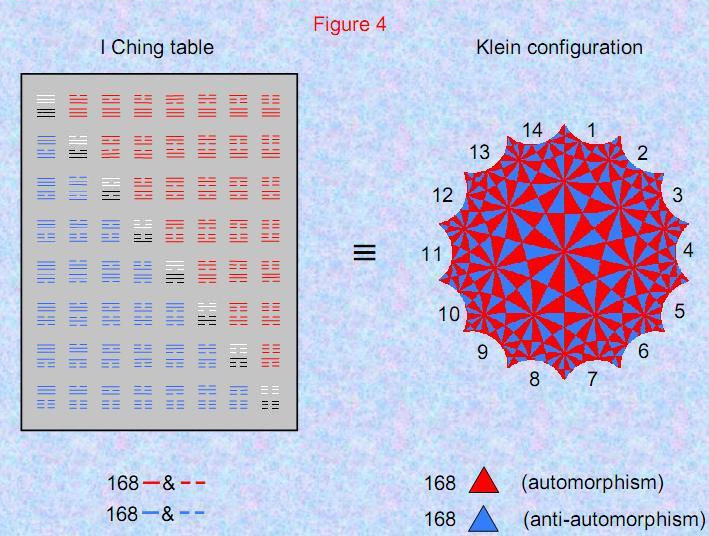9

Figure 5

In the {3,7} tiling of the Riemann surface of the Klein quartic, 56 triangles with 168 vertices tessellate completely the hyperbolic plane. The vertices denote the 168 automorphisms of the Klein quartic. The triangles correspond in the I Ching table to the 56 red trigrams on one side of the diagonal with 168 lines and broken lines, each one corresponding to an automorphism of this equation well-known to mathematicians.

10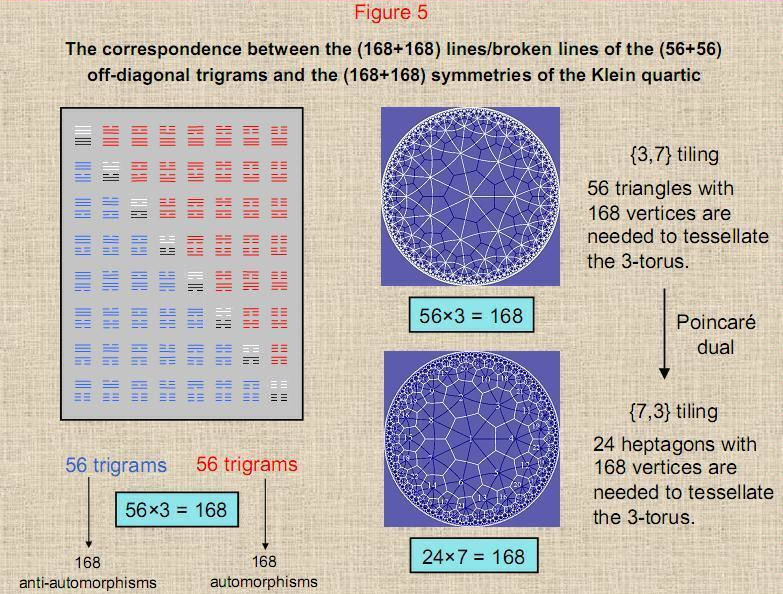11

Figure 6

The {3,7} tiling onto the 3-torus of the 168 automorphisms of the Klein quartic represented by the 168 vertices of the 56 triangles of the Klein configuration requires seven colours to colour the 56 triangles so that no two triangles sharing an edge have the same colour. The 3-torus may be deformed into a tetrahedral structure formed by sticking the 12 square faces of six square antiprisms onto the 12 square faces of four triangular prisms and then twisting each antiprism. This creates 56 triangles (eight triangular faces of the four prisms + 48 triangular faces of the six square antiprisms). The 56 triangular faces define the 56 vertices of seven cubes. As a cube has 24 different rotations, the 168 automorphisms of the Klein quartic correspond to seven copies of 24 symmetries of the octahedral group. Its 168 anti-automorphisms are represented by the 168 vertices of the 56 triangular faces of the tetrahedral structure after it has been turned inside out. These triangles, too, define the 56 vertices of seven cubes that generate seven copies of the 24 symmetries/inversions of the octahedral group. These make up the 168 anti-automorphisms of the Klein quartic.

12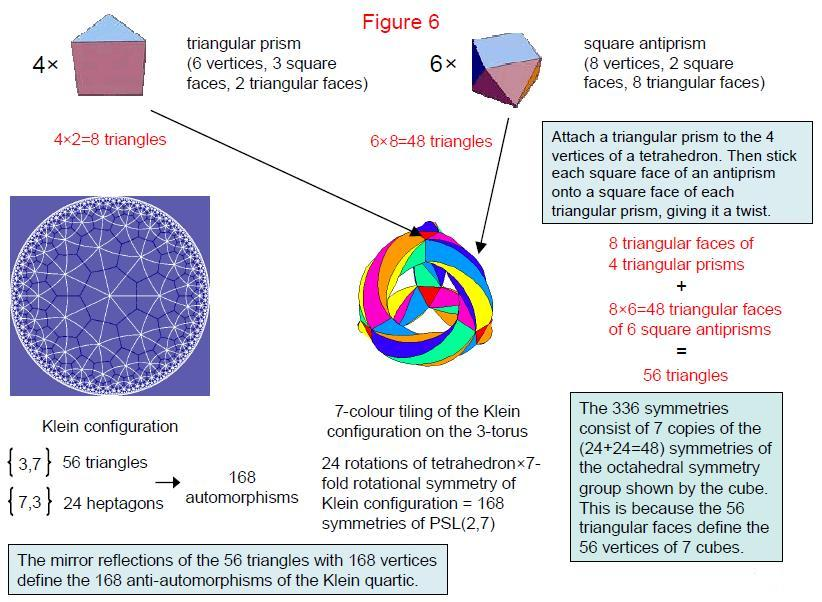13

Figure 7

The 168 automorphisms of the Klein quartic belong to PSL(2,7). This group is isomorphic to SL(3,2), whose 168 symmetries are displayed by the Fano plane, the simplest projective plane. Implicit in its geometry is a Golden Rectangle, the ratio of whose adjacent sides is the Golden Ratio φ = 1.6180..., and a Golden Rhombus, the lengths of whose diagonals are in the same proportion. The 60 edges of a rhombic triacontahedron form 30 Golden Rhombi that are the bases of pyramids with four faces, creating the 120 faces of the disdyakis triacontahedron. This shows how the ideal, aesthetically pleasing proportion of the Golden Ratio, used throughout history by artists and architects, manifests in the form of the disdyakis triacontahedron. Beauty is exhibited in the shape, as well as in the mathematical properties, of the polyhedral version of the inner Tree of Life.

14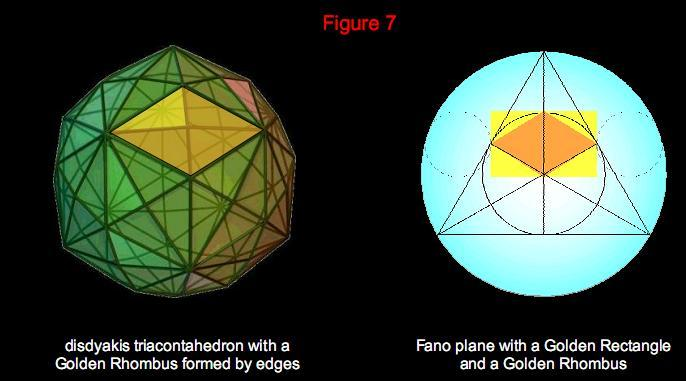The Fano plane has the 168 symmetries of SL(2,3), which is isomorphic to PSL(2,7), the group of symmetries of the Klein quartic.        The Golden Rhombic shape of the faces of the rhombic triacontahedron underlying the disdyakis triacontahedron is implicit in        the geometry of the Fano plane, which represents the algebra of the octonions.

15

Figure 8

A continuous, logical link connects the (2,3) torus knot to the quantitative description given in 1908 by Annie Besant and C.W. Leadbeater of the form of the heterotic superstring constituent of up and down quarks.

16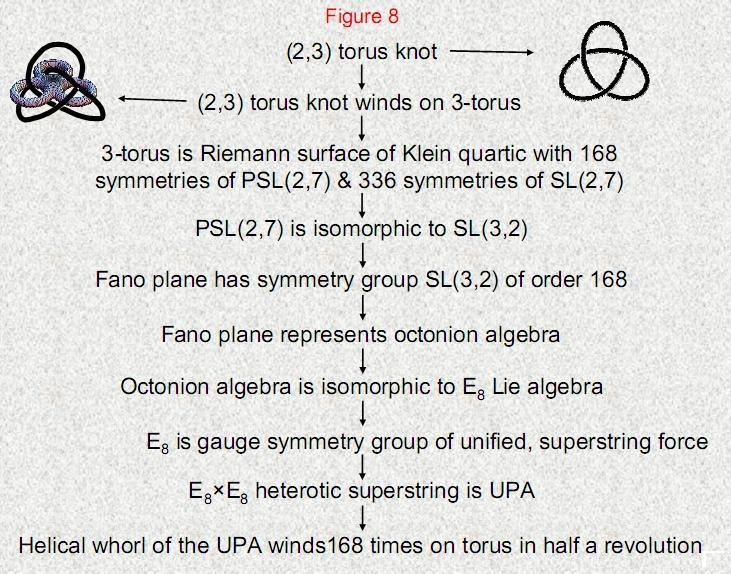17

Figure 9

The polyhedral inner form of the Tree of Life is the 144 Polyhedron, which has 74 vertices, 216 edges and 144 faces. It generates another polyhedron with 62 vertices, 180 edges and 120 triangular faces. The latter is the disdyakis triacontahedron and the former is the disdyakis dodecahedron with tetrahedra added to its 48 faces. Their (24+24=48) peaks correspond to the 24 rotational symmetries of the octahedral group and the 24 rotations/inversions. This is a subgroup of SL(2,7), and seven copies of it define the 336 symmetries of SL(2,7), the double-cover group of PSL(2,7), which is isomorphic to SL(2,7)/Z2 and describes the 168 automorphisms of the Klein quartic. This symmetry manifests in the 168 edges of the disdyakis triacontahedron above and below the equator that is perpendicular to the axis joining two diametrically opposite A vertices of this polyhedron. The centre of PSL(2,7) is Z3, the cyclic group of order 3 that is isomorphic to the three primitive roots of 1, namely, 1, exp(2πi/3) & exp(4πi/3). The fact that the dimension of SL(2,7) is the number of edges above and below the equator of the disdyakis triacontahedron is evidence that PSL(2,7) is embodied in this polyhedron as a fundamental symmetry.

The algebra of the seven unit imaginary octonions is represented by the Fano plane, which has the symmetry of SL(3,2), a group that is isomorphic to PSL(2,7). It can alternatively be represented by assigning them to the vertices and centre of an octahedron. This suggests a connection between the disdyakis triacontahedron and the octonions. It is to be expected because the octonions form a basis of E8, the superstring gauge symmetry group (see Figs. 7 & 8).

18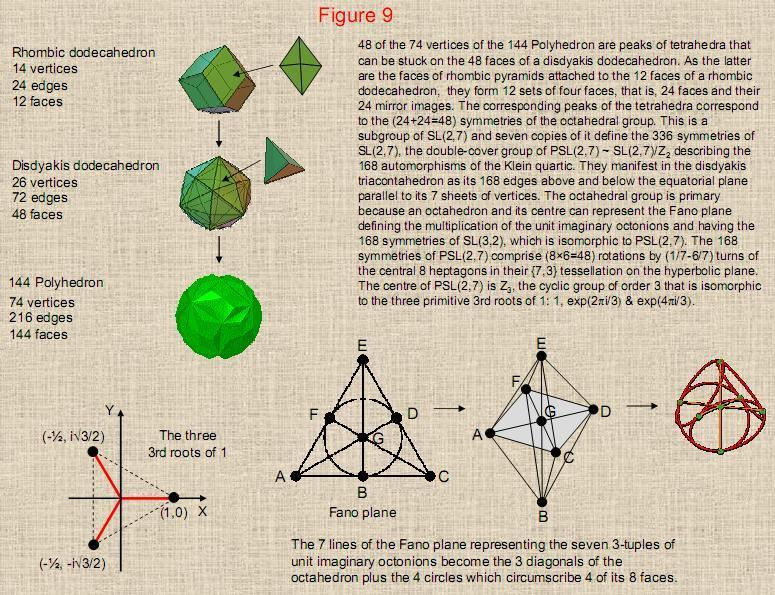19

Figure 10

If a Yang (unbroken) line of a trigram denotes a face of a cube orientated along one of the three perpendicular, positive directions and a Yin (broken) line denotes a face facing a negative direction, the eight trigrams symbolize the sets of three perpendicular faces that intersect at the eight corners of a cube. As a pair of different trigrams, a hexagram defines the straight line joining two corners of a cube. The pairs of identical trigrams in the eight hexagrams in the diagonal of the I Ching table define the eight corners themselves. The ordering of pairs of trigrams signifies the directions of the lines regarded as arrows. This means that the hexagrams below the diagonal signify arrows connecting different corners that point in the opposite direction to those connecting corners signified by hexagrams above the diagonal. The 28 hexagrams above the diagonal have 168 lines/broken lines denoting the 168 faces (84 positive, 84 negative) defined by the endpoints of the 28 lines joining different corners. Each combination of three faces appears seven times, creating seven copies of each corner, i.e., 56 copies of the eight corners, totalling 64 corners. These are the counterparts of the 56 hyperbolic triangles with 168 vertices in the {3,7} tiling of the automorphisms of the Klein quartic on the hyperbolic plane, each triangle in fact defining one of the corners of seven cubes. The 56 hyperbolic triangles mapped onto a 3-torus turned inside-out, whose 168 vertices denote the 168 anti-automorphisms of the Klein quartic, similarly correspond to the 56 trigrams with 168 lines/broken lines in the 28 hexagrams below the diagonal, each trigram defining a corner of seven copies of a cube - the seven cubes whose 56 corners are defined by these 56 triangles. The Yang/Yin nature of each line/broken in the I Ching table signifies whether the faces of the cube are orientated towards the positive or negative directions of each perpendicular axis. These 168 bi-polar degrees of freedom manifest in the disdyakis triacontahedron as the 168 edges other than the 12 edges in its equatorial plane perpendicular to an axis joining two diametrically opposite A vertices. Regarded as arrows that can point in two opposite directions, the edges of the polyhedron point in 168 directions above the equatorial plane and 168 directions below it. The 168 pairs of oppositely directed edges are the counterpart of the 168 automorphisms and the 168 anti-automorphisms of the Klein quartic and the 168 lines and 168 broken lines in the 56 off-diagonal hexagrams of the I Ching table.

20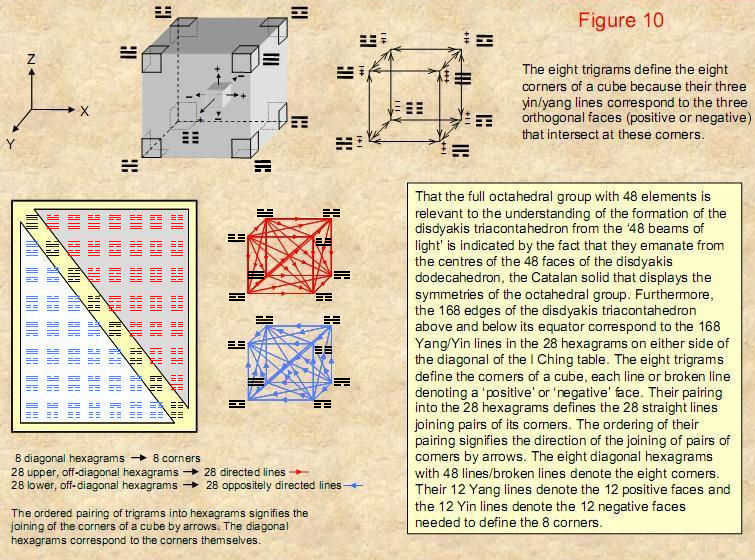21

Figure 11

In its {7,3} tiling, the Klein configuration (KC) is divided into seven identical sectors. A sector is composed of two half-sectors, each with 12 hyperbolic triangles, so that KC is divided into 14 sectors with 168 triangles. Compare this with the 14 triangles in the fourth layer of the Sri Yantra. When each triangle is constructed from three tetractyses, there are 12 hexagonal yods on their six edges. This means that (14×12=168) hexagonal yods line on the (14×6=84) edges of the (14×3=42) tetractyses comprising the 14 triangles. KC is isomorphic in this sense to the last layer of triangles of the Sri Yantra. To establish such isomorphism, however, we need to consider only each triangle as a tetractys, for 168 yods symbolizing the 168 automorphisms line the 63 edges of the 21 triangles in one half of the Sri Yantra and 168 yods symbolizing the 168 anti-automorphisms line the 63 edges of the 21 triangles in its other half. Unlike, however, in the case of the disdyakis triacontahedron, one half of the Sri Yantra is not the exact mirror image of its other half.

22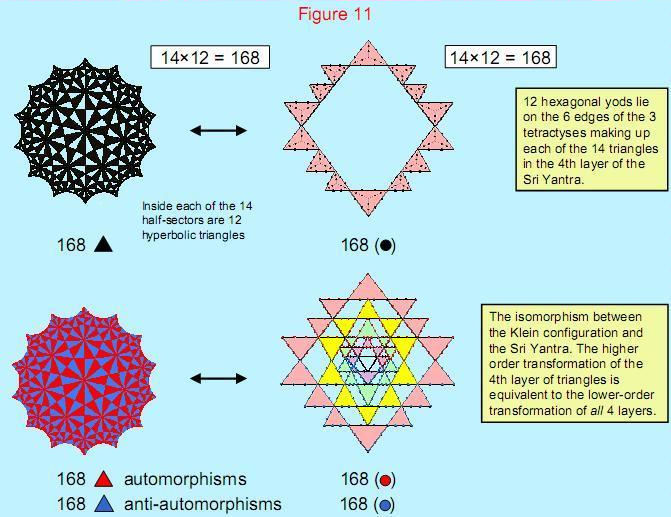23

Figure 12

It was shown in Part 2 (pp. 21, 22) that the Sri Yantra and the lowest seven overlapping Trees of Life are equivalent. Here is displayed the isomorphism between the Sri Yantra and the inner form of seven Trees of Life – or rather, the first six polygons enfolded in each tree, as these two sets of polygons constitute a Tree of Life pattern in themselves. There are 168 corners associated with the 42 polygons enfolded in seven overlapping Trees of Life on each side of its central pillar. They correspond to the 168 yods lying on edges of the 21 triangles in each half of the Sri Yantra. The 42 (black) vertices associated with each set of 21 triangles correspond to the 42 (black) corners associated with the squares and hexagons in each set of six polygons. The 42 (red) hexagonal yods on edges of each set of seven triangles in the fourth layer correspond to the 42 (red) corners of the octagons outside their shared edges in each set of polygons. The 84 (blue) hexagonal yods on the 42 edges of the 14 triangles in each half of the first three layers correspond to the 84 (blue) corners of the triangle, pentagon & decagon in each set of polygons outside their root edges.

24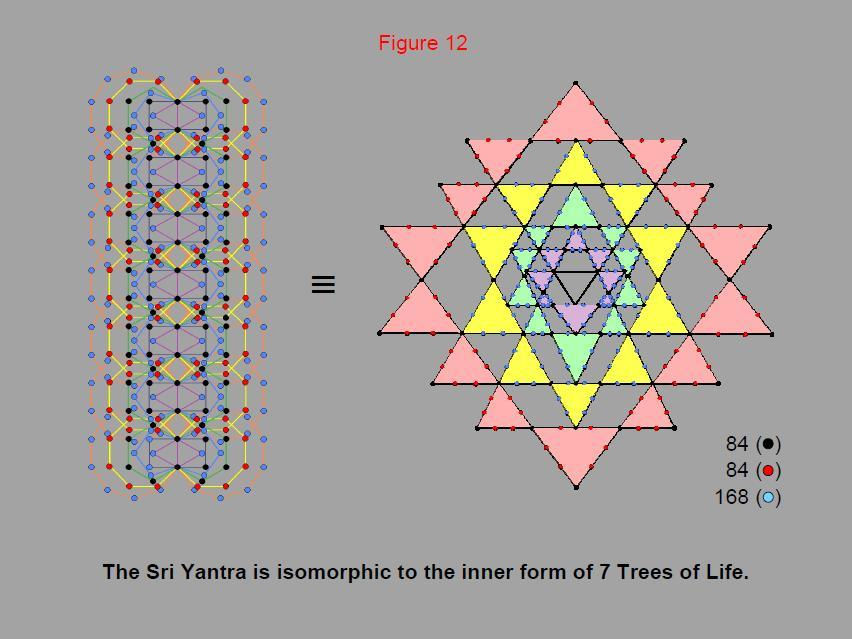25

Figure 13

Of the 384 yods in the tetractyses forming the lowest seven Trees of Life up to the top of the seventh tree, 48 (red) yods are in the lowest tree up to the level of Chesed, the first Sephirah of Construction. The counterpart of this in the Sri Yantra is that there are 48 (red) yods which are either centres of triangles or hexagonal yods on edges of the central triangle. The 336 (black) yods on the edges of the 42 triangles correspond to the 336 (black) yods in the lowest seven trees above Chesed of the lowest tree.

26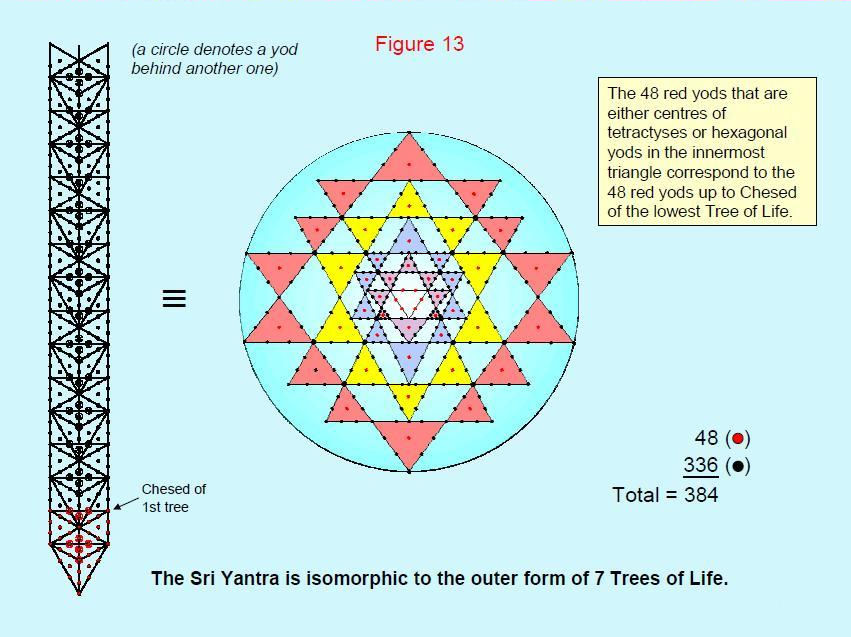27

Figure 14

This displays the corresponding features of the inner Tree of Life, the I Ching table and the Sri Yantra as isomorphic representations of holistic systems.

1. The six green yods either at the external corners of the triangles or at the topmost and lowest corners of the hexagons coincide with Sephiroth. They correspond to the six lines of the pair of Heaven trigrams in the top left-hand corner of the table and to the six green hexagonal yods on the edges of the central triangle of the Sri Yantra.

2. The 21 black yods denoting corners of one set of the first six polygons that lie outside their shared edge correspond to the 21 black lines/broken lines in the upper trigrams of the seven remaining diagonal hexagrams and to the black centres of the 21 triangles in one half of the Sri Yantra. The 21 white yods denoting external corners of the other set of polygons correspond to the 21 white lines/broken lines in the lower trigrams of the seven hexagrams on the diagonal of the table and to the white centres of the 21 triangles in the other half of the Sri Yantra.

3. The 168 red yods in one set of six polygons that are not corners correspond to the 168 red lines/broken lines in the 28 off-diagonal hexagrams of half of the table and to the 168 red yods on the 63 edges of the 21 triangles in one half of the Sri Yantra. The 168 blue yods in the other set of six polygons that are not corners correspond to the 168 blue lines/broken lines in the 28 off-diagonal hexagrams of the other half of the table and to the 168 blue yods on the 63 edges of the 21 triangles in the other half of the Sri Yantra.

28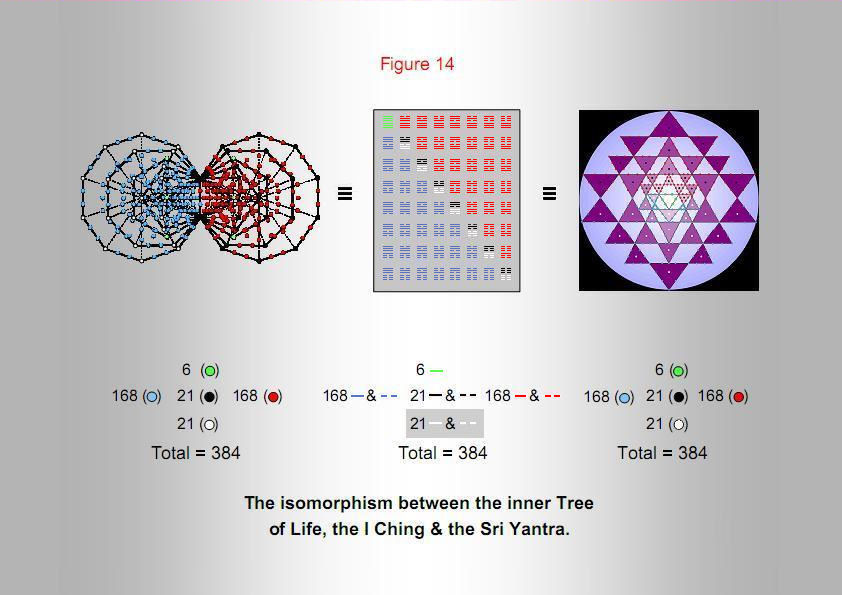29

Figure 15

192 yods are associated with each set of the first six enfolded polygons of the inner Tree of Life. They are intrinsic to that set because none of them is shared with the six polygons enfolded in the next higher Tree of Life, which also have 192 such yods. The 192 yods associated with each set of six polygons correspond to the 192 lines/broken lines in each half of the I Ching table.

30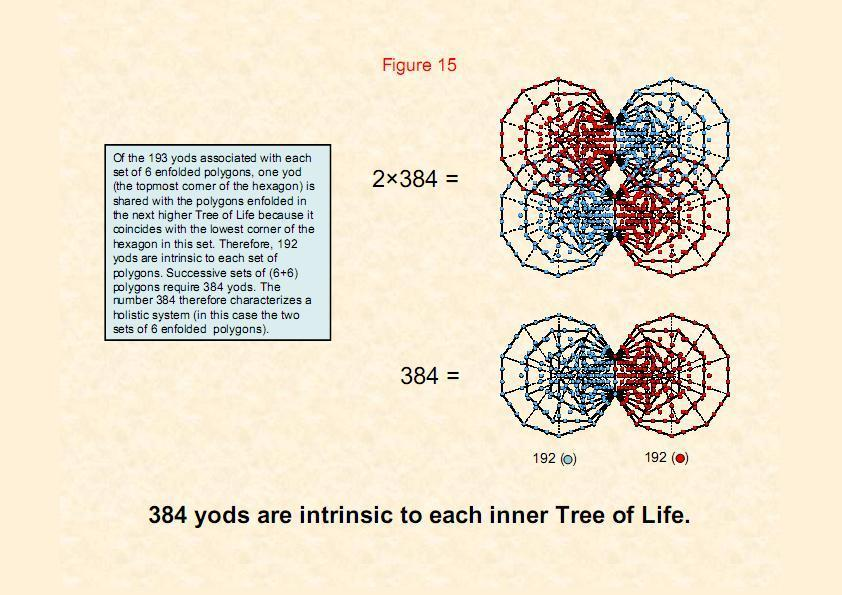31

Figure 16

Considered as tetractyses, the 21 triangles in one half of the Sri Yantra have 21 black centres and 21 white pairs of vertices, i.e., 21 triplets of one white yod and two black yods. They also have 21 purple triplets of hexagonal yods and 21 brown triplets of hexagonal yods, each pair of triplets forming a Star of David. The 189 yods associated with the 21 tetractyses in each half of the Sri Yantra comprise three sets of 21 triplets of yods, i.e., 63 triplets.

The 21 centres of tetractyses in each half of the Sri Yantra comprise 12 centres of the tetractyses in the third and fourth layers and nine centres of tetractyses in the first and second layers. The significance of this will be discussed on pages 41 & 42.

32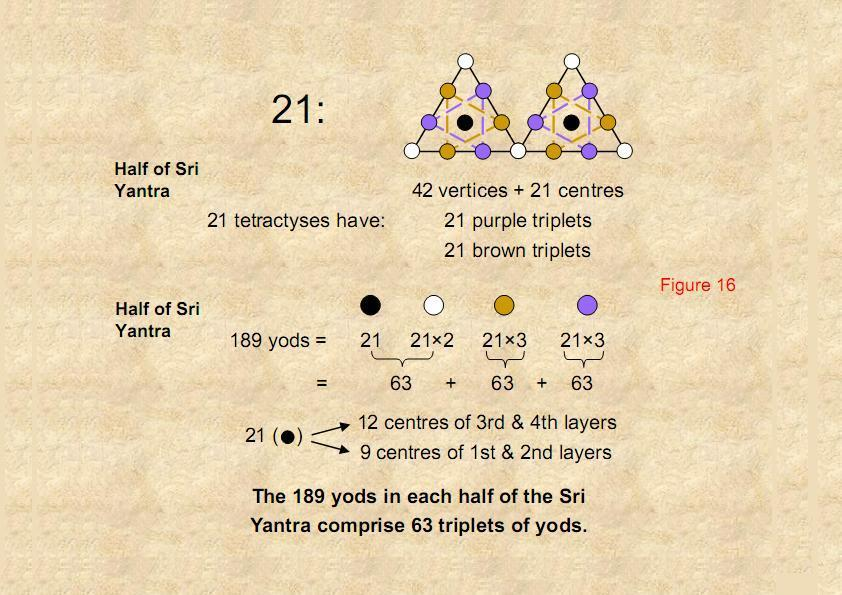33

Figure 17

Selection of successive notes of the Pythagorean scale as the starting note (tonic) generates seven possible musical scales. These sequences of intervals (T) and leimmas (L), the counterpart of the modern semitone, define the musical modes of the Roman Catholic Church. The four “authentic modes” are the Dorian mode (D scale), the Phrygian mode (E scale), the Lydian mode (F scale) & the Mixolydian mode (G scale). They have four “hypo” modes separated by the interval of a perfect fourth from their authentic counterparts – the Hypodorian (A scale), the Hypophrygian (B scale), the Hypolydian (C scale) & the Hypomixolydian (D scale). In terms of notes, the lattermost is identical to the Dorian, differing in the choice of the ‘dominant’ and ‘reciting note.’

The table displays the ‘tone ratios’ of the notes in the seven musical scales. These are the frequencies or pitches of the notes relative to that of the tonic. The Pythagorean musical scale is the C scale (Hypolydian mode). Tone ratios of notes belonging to this scale are written in black; non-Pythagorean tone ratios are written in red. The seven scales have 26 Pythagorean notes between the tonic and the octave, showing how YAHWEH, the Godname of Chokmah with number value 26, prescribes the seven musical scales. Their notes have 14 different tone ratios, i.e., there are 12 distinct types of notes between the tonic and octave. The sequence of 14 notes is split into the first seven, primary notes and their seven “complements” (notes whose tone ratios are the interval between its corresponding primary note and the octave). They comprise the eight notes of the Pythagorean scale and six non-Pythagorean notes. The 12 types of notes between the tonic and octave comprise six Pythagorean notes and six non-Pythagorean notes.

34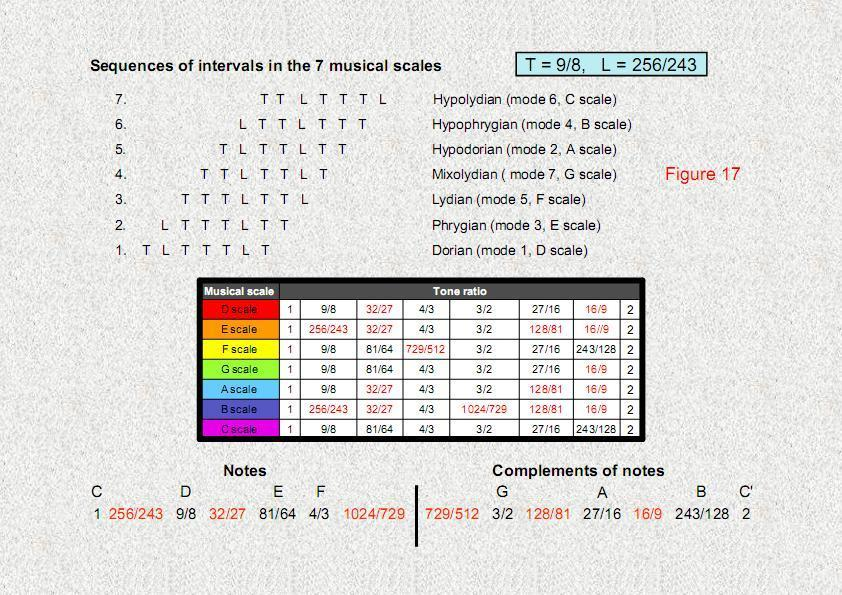35

Figure 18

There are 28 intervals between the eight notes of a musical scale. Excluding the octave, there are (7×27=189) intervals between the notes of the seven varieties of scales. They all have the values of the tone ratios of the 12 types of notes between the tonic and the octave found in the seven scales. The second table lists the number of intervals of each type. The number in the brackets next to each one is the number of times the note with that tone ratio appears in the scales. Some of the notes and intervals can be paired with their complements. Their numbers are listed in the third table, together with the numbers of intervals without complements. There are 21 notes and 42 intervals that have complements. They form two sets of 63 intervals, one set paired with its complementary set, creating 63 pairs. There are 63 unpaired intervals. The 189 intervals therefore divide into three sets of 63 intervals, that is, 63 triplets, each triplet comprising a pair of notes or intervals and an unpaired interval. They comprise 21 notes made of the second, third & fourth notes of the seven scales and 168 intervals made up of the 21 complements of these notes, 42 intervals and their 42 complements and 63 unpaired intervals.

36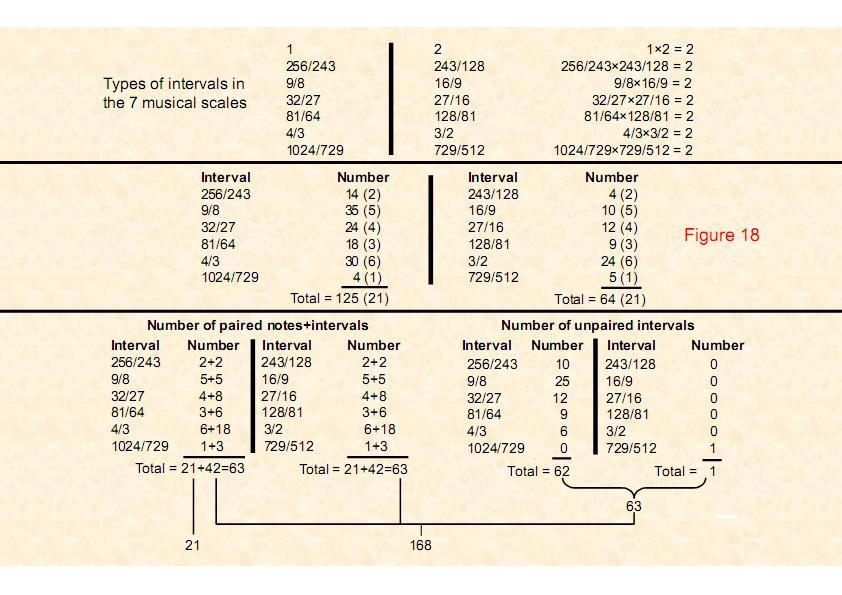37

Figure 19

Between the notes of the seven scales, there are 189 rising intervals below the octave. They also have 189 falling intervals (this is the interval between the lower note and the higher note. It is the reciprocal of the corresponding rising interval). So there are 378 rising and falling intervals other than the octave. As the seven scales constitute a holistic system, their patterns of intervals are encoded in the inner form of the Tree of Life. The two sets of the first six enfolded polygons have 384 yods that are intrinsic to them. Six of them coincide with Sephiroth or Daath, leaving 378 yods that do not belong to the outer form of the Tree of Life. The 189 yods in one set of polygons denote rising intervals. Their mirror images in the other set denote falling intervals.

As evidence that this is not a coincidence, the yod populations of pairs of polygons correlate exactly with the numbers of different classes of intervals. The 21 corners of polygons that are outside their shared edge and which do not coincide with Sephiroth of the Tree of Life symbolize the 21 notes made up of the second, third & fourth notes of each scale. Their mirror images in the other set of polygons denote the complements of these notes (not necessarily in the same scale, of course). The 21 corners comprise 12 corners of the square, hexagon & decagon, and nine corners of the pentagon & octagon. The 42 yods in the square & hexagon symbolize the 42 intervals that are not notes. The 63 yods in the triangle & decagon denote the 63 complements of the notes and intervals. The 63 yods in the pentagon & octagon denote the 63 intervals lacking a complement.

This is the Tree of Life basis of the seven types of musical scales as a holistic system.

38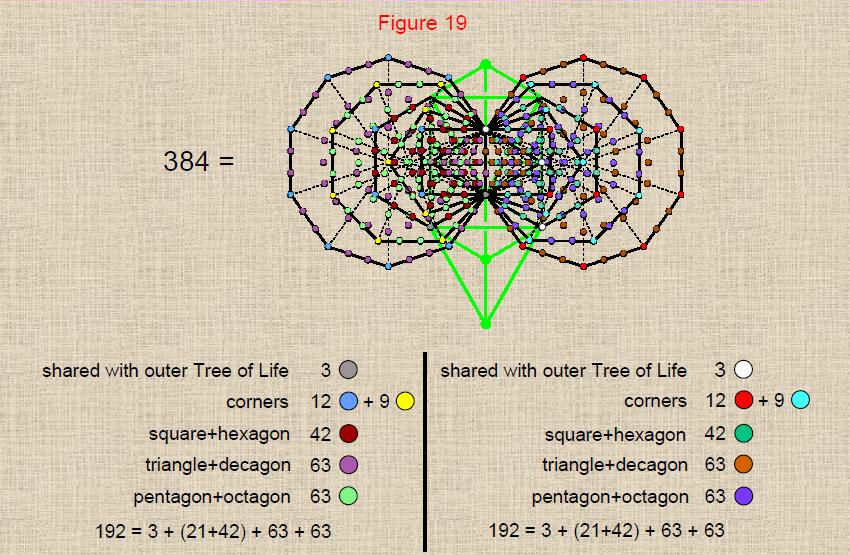Tree of Life representation of the three sets of 63 rising & falling intervals between the notes of the seven musical scales. 39

Figure 20

This correlation between the intervallic structure of the seven musical scales and the sacred geometry of the inner Tree of Life extends to the tetractys-transformed Sri Yantra as well. The 21 notes made up of the first three notes above the tonic in each scale determine the seven scales collectively because the 21 remaining notes below the octave are their complements and therefore are defined by them. They are symbolized as rising intervals by the yods at the centres of the 21 triangles/tetractyses in one half of the Sri Yantra, the centres of the 21 tetractyses in the other half denoting their corresponding 21 falling intervals (note: not their complements) . The tips of the 21 triangles denote the 21 complements of the notes. The tips of the 21 triangles in the other half of the Sri Yantra denote their falling interval counterparts. The 42 intervals and their complements are symbolized by the 42 hexagonal yods on each of the two edges of each triangle forming its tip. Their corresponding falling intervals are symbolized by the counterparts of these yods in the other half of the Sri Yantra. The 63 intervals without complements are symbolized by the 63 yods on the bases of the 21 tetractyses in one half, their falling interval counterparts being denoted by the 63 yods on the bases of the 21 tetractyses in the other half of the Sri Yantra.

The centres of the 21 tetractyses in either half of the Sri Yantra comprise the centres of the 12 tetractyses in the third and fourth layers and the centres of the nine tetractyses in the first and second layers. This 12:9 differentiation is the counterpart of the distinction between the 12 notes above the tonic made up of the second, third & fourth notes in the four authentic modes and the nine notes above the tonic made up of the second, third & fourth notes in the three plagal modes (the fourth, plagal mode is not a distinct octave species, the Hypomixolydian mode having the same sequence of notes as the Dorian). The uppermost first and second layers of the Sri Yantra therefore correlate with the three plagal modes that have different sets of notes, whilst its third and fourth layers correlate with the four authentic modes.

40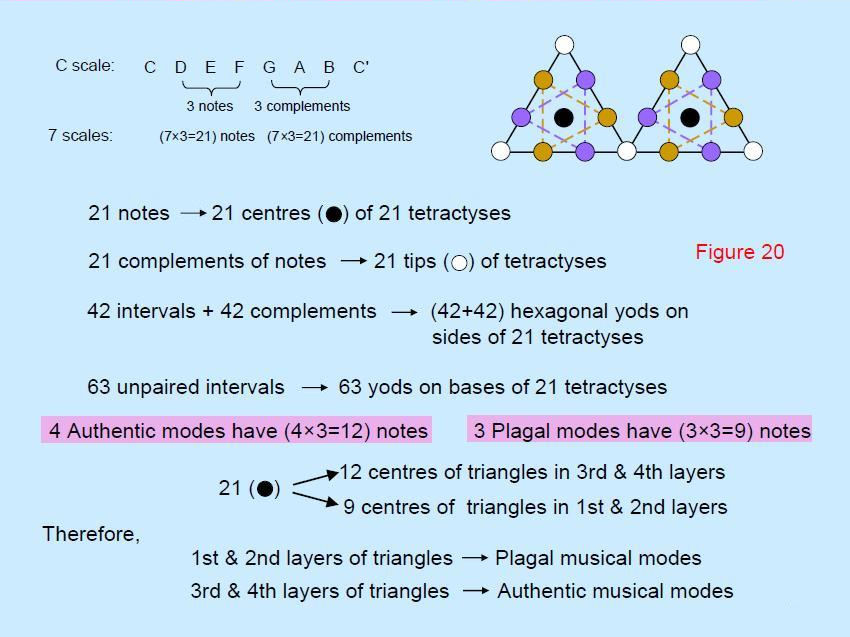41

Figure 21

Arranged in a Star of David in each tetractys of the Sri Yantra, the two triads of hexagonal yods correspond to the two trigrams in each hexagram of the I Ching table. The six green, unbroken lines of the two Heaven trigrams at the upper left-hand corner of the table correspond to the six hexagonal yods arranged as two triangles in a Star of David in the central triangle (see Fig. 14). The (21+21) black lines/broken lines of the seven remaining hexagrams in the diagonal correspond to the centres of the two sets of 21 tetractyses. The 84 white lines/broken lines of the 14 hexagrams containing the Heaven trigram correspond to the 84 corners of the 42 tetractyses. Each category ‘marks out’, so to speak, the boundary of their respective systems. The 21 purple trigrams on one side of the diagonal correspond to the 21 triplets of purple, hexagonal yods in one half of the Sri Yantra. The 21 purple trigrams on the other side of the diagonal correspond to the 21 triplets of purple, hexagonal yods in the other half of the Sri Yantra. Similarly for the brown, hexagonal yods. The I Ching table is a non-geometrical version of the Sri Yantra.

42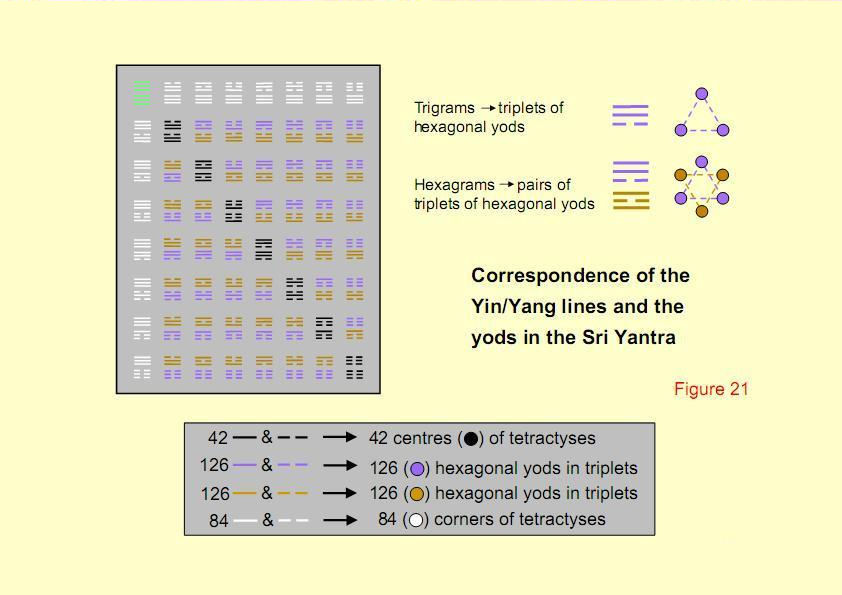43

Figure 22

Displayed here is the detailed correspondence between different types of trigrams in the I Ching table and the triplets of hexagonal yods in the tetractys-transformed triangles of the Sri Yantra. Lines/broken lines and their counterpart yods have the same colour.

44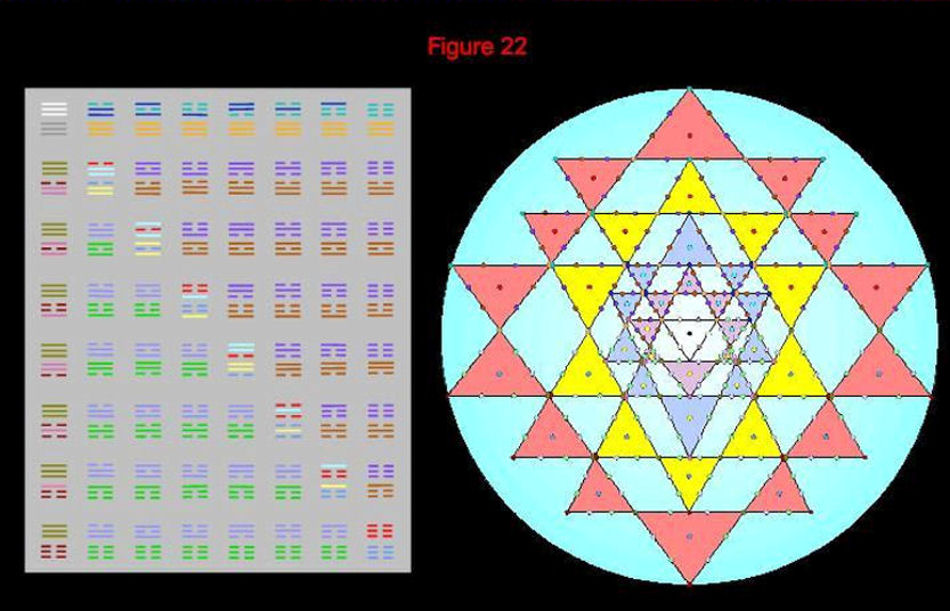Correspondence between the I Ching table and the Sri Yantra. 45

Figure 23

The seven separate, regular polygons constituting the inner form of the Tree of Life comprise 295 yods when their 48 sectors are turned into tetractyses. In other words, starting with the seven polygons divided into their sectors, (295-48-7=240) more yods are needed to transform their sectors into tetractyses. There are 48 centres of tetractyses. This leaves (240-48=192) hexagonal yods arranged at the corners of six-sided polygons (i.e., at the tips of Stars of David). 192 yods are similarly arranged in the other set of polygons. In terms of the formal equivalence between the Tree of Life and the tetractys, the six yods at the corners of a six-sided polygon (it does not need to be a hexagon, of course) symbolize the six Sephiroth of Construction above Malkuth. These two sets of 192 hexagonal yods arranged at the corners of triangles are the Tree of Life counterpart of the 192 lines/broken lines grouped in trigrams in each half of the I Ching table. The 24 red lines/broken lines in the upper trigrams of the eight diagonal hexagrams correspond to the 24 red, hexagonal yods in one of the hexagons. The 24 blue lines/broken lines in the lower trigrams of these eight hexagrams correspond to the 24 blue, hexagonal yods in the hexagon belonging to the other set of polygons. As 24 = 4×3×2 and as each sector of a polygon contributes four of these hexagonal yods, the counterpart of the two sets of four diagonal trigrams are the four hexagonal yods per sector multiplied, firstly, twice (a hexagon is two equilateral triangles rotated by 60°) and then thrice (pairs of hexagonal yods are arranged at each corner of a triangle). The trigrams are expressing the three- fold, rotational symmetry of an equilateral triangle – a sector of a hexagon. The eight-fold nature of trigrams corresponds to the fact that there are eight hexagonal yods on the edges of a pair of adjacent sectors in the hexagon. These are basic because the rotation of each one by 120° about the centre of the hexagon generates all its remaining yods.

46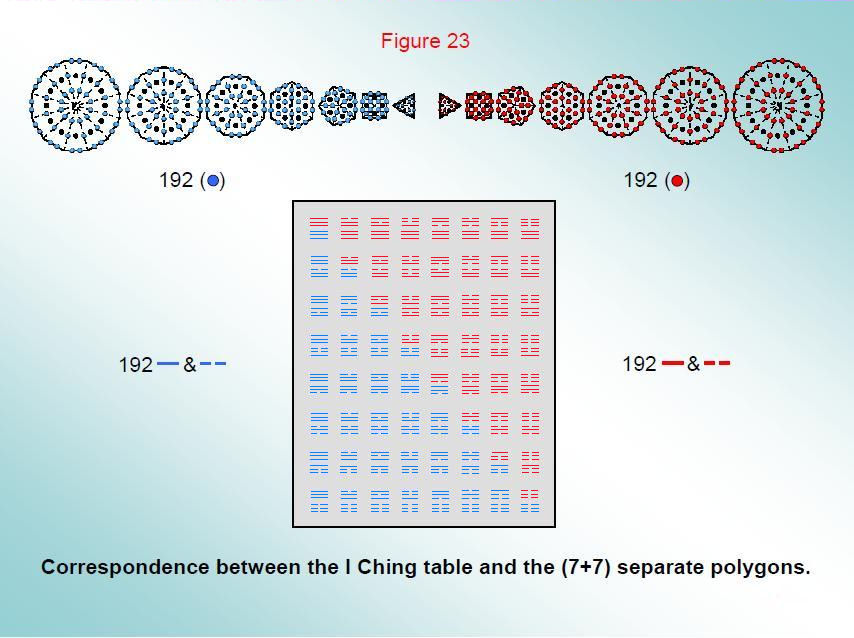47

Figure 24

Divided into their sectors, the seven separate polygons have 48 corners, 96 edges and 48 triangles surrounding their centres, i.e., 192 geometrical elements. They correspond to the 192 lines/broken lines in one diagonal half of the I Ching table. The 192 geometrical elements in the other set of seven polygons of the inner Tree of Life correspond to the 192 lines/broken lines in the other half of the table. The 12 lines and 12 broken lines in the upper trigrams of the eight diagonal hexagrams correspond to the 12 edges and 12 corners/triangles of the sectors of the hexagon. Similarly, the 12 lines and 12 broken lines in the lower trigrams correspond to the 12 edges and 12 corners/triangles of the sectors of the hexagon in the other set of polygons. The 384 lines and broken lines in the table express the number of geometrical elements needed to construct the two sets of seven polygons making up the inner form of the Tree of Life. They symbolize the independent ‘bits of information’ needed to build a holistic system.

48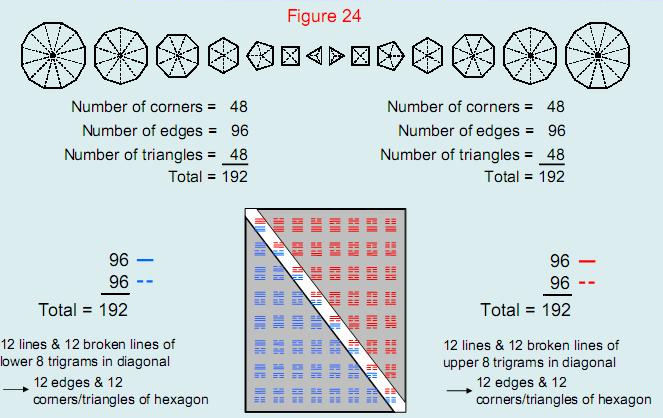The 384 lines & broken lines of the I Ching table denote the 384 geometrical elements composing the 14 regular polygons of the inner Tree of Life.

49

Home